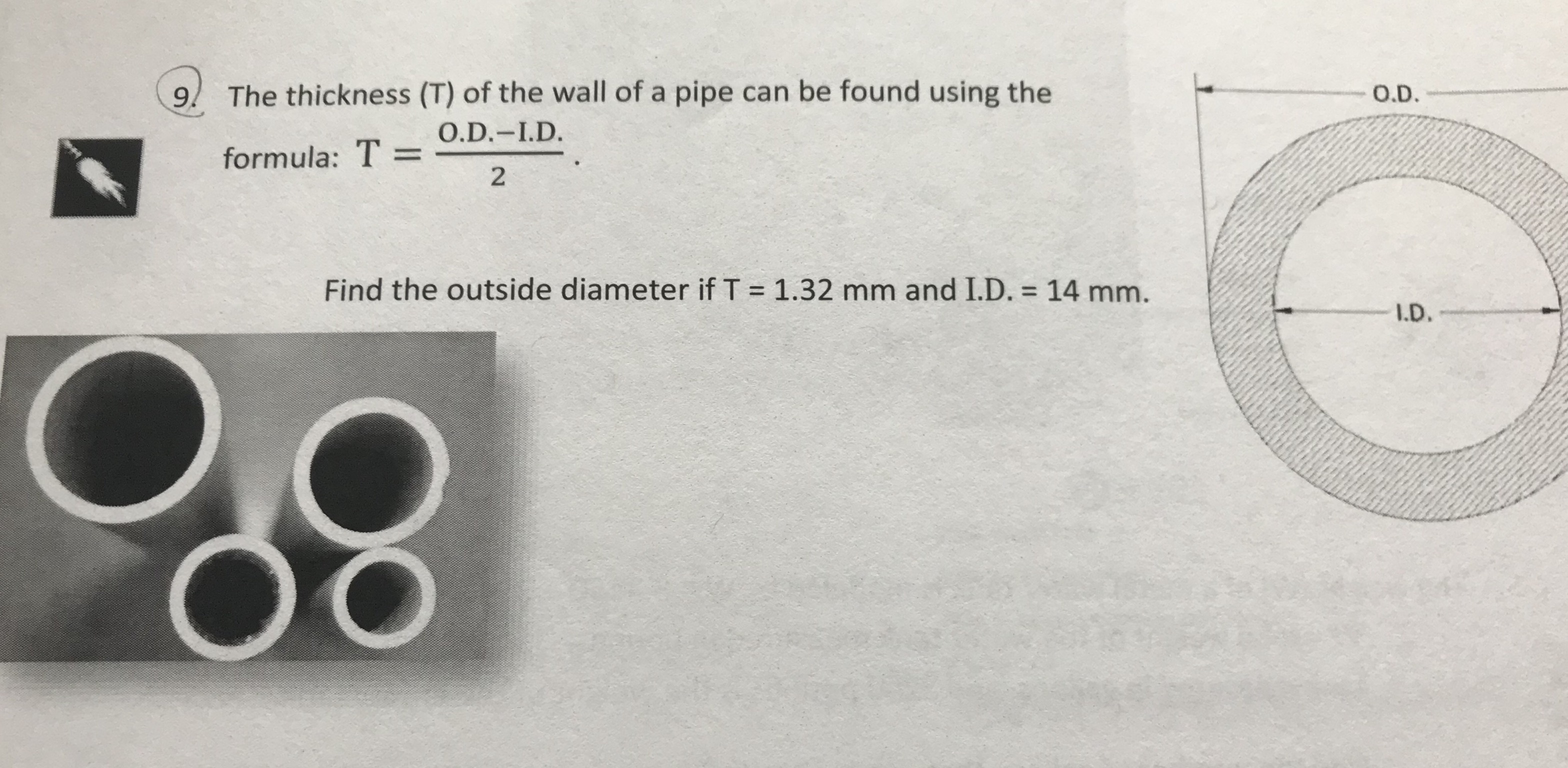# 9! The thickness (T) of the wall of a pipe can be found using theO.D.formula: T = OD-ID.O.D.-I.D.2Find the outside diameter if T = 1.32 mm and ID. = 14 mm.I.D.

Question
77 views

Please provide detailed (step by step) solutionhelp_outlineImage Transcriptionclose9! The thickness (T) of the wall of a pipe can be found using the O.D. formula: T = OD-ID. O.D.-I.D. 2 Find the outside diameter if T = 1.32 mm and ID. = 14 mm. I.D. fullscreen
check_circle

Step 1

Introduction:

Denote the thickness of the wall of the pipe as T.

Denote the outside diameter of the wall of the pipe as O.D.

Denote the outside diameter of the wall of the pipe as I.D.

The general formula to find the thickness of the wall of a pipe is,

Step 2

Obtain the outside diameter of the wall of a pipe:

The thickness of the wall of a pipe is given as T = 1.32 mm.

The inside diameter of the wall of a pipe is given as I.D = 14 mm.

Th...

### Want to see the full answer?

See Solution

#### Want to see this answer and more?

Solutions are written by subject experts who are available 24/7. Questions are typically answered within 1 hour.*

See Solution
*Response times may vary by subject and question.
Tagged in